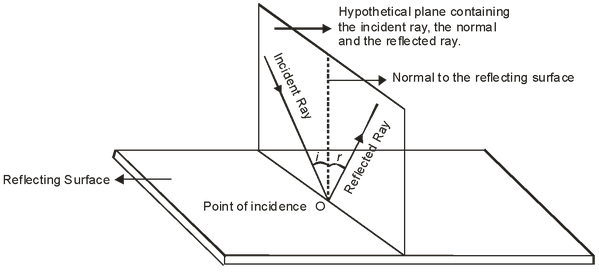# Laws Of Reflection

## Light of Class 10

### LAWS OF REFLECTION

There are two laws of reflection

### FIRST LAW OF REFLECTION:

According to the first law of reflection, the incident ray, the reflected ray and the normal at the point of incidence all lie in the same plane which is perpendicular to the plane of the reflecting surface.### SECOND LAW OF REFLECTION:

According to the second law of reflection, the angle of incidence is always equal to the angle of reflection for small angles.

i.e. ∠i = ∠r

Where ∠i = angle of incidence.

∠r = angle of reflection.

The laws of reflection of light apply to all kinds of mirrors, plane mirrors as well as spherical mirrors.

• A ray of light which is incident normally on a reflecting surface (say a mirror), is reflected back along the same path because the angle of incidence as well as the angle of reflection for such a ray of light are zero. (i.e. ∠ i = ∠ r = 0°.)

Ex. What happens a ray of light falls normally (or perpendicularly) on the surface of a mirror?

Solution: A ray of light which is incident normally on a mirror, is reflected back along the same path because the angle of incidence as well as angle of reflection for such a ray of light are zero.